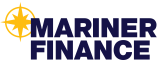# Calculate Simple Interest using a Loan calculator

##Interest rate calculator for simple interest loan

Confused about your interest charge? If you have an interest-bearing loan, or what is sometimes referred to as a simple interest loan, Web math created an interest rate calculator that breaks down the formula to help simplify the calculation.

## How does a loan calculator factor your interest?

Great question, the formula loan calculators use is I = P * r *T in layman’s terms Interest equals the principal amount multiplied by your interest rate times the amount in years.

For example

Where:

• P is the principal amount, \$3000.00.
• r is the interest rate, 4.99% per year, or in decimal form, 4.99/100=0.0499.
• t is the time involved, 3….year(s) time periods.
• So, t is 3….year time periods.

To find the simple interest, we multiply 3000 × 0.0499 × 3 to get that:

 The interest is: \$449.10

Usually, now, the interest is added to the principal to figure some new amount after 3 year(s),
or 3000.00 + 449.10 = 3449.10. For example:

• If you borrowed the \$3000.00, you would now owe \$3449.10
• If you loaned someone \$3000.00, you would now be due \$3449.10
• If owned something, like a \$3000.00 bond, it would be worth \$3449.10 now.

Click here to use this helpful loan calculator and use it as a reference when calculating simple interest.

## How do you calculate a loan payment?

It’s understandable you may want to determine if a loan is affordable and a loan calculator or a bit of math can help you find out what your monthly payments may be. Calculators are great for quick answers so let’s look into how to calculate your monthly loan payment with a monthly payment calculator.

However to do that first you must identify which type of loan you have.

There are two types, interest-only loans where you only service the loan by paying interest and amortizing loans which you pay down the loan balance over a set period of time.

For simply interest loans you can use this loan calculator.

For amortizing loans you can use this personal loan calculator.

If you’re interested in a personal loan today you can apply here at Mariner Finance.

Still confused? Contact your local Mariner Finance branch today to speak with a knowledgeable staff member about your account.

[/fusion_text][/fusion_builder_column][/fusion_builder_row][/fusion_builder_container][fusion_global id=”3780″]